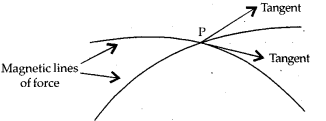# Why not two magnetic lines of force intersect each other?

Why not two magnetic lines of force intersect each other?

The tangent at any point on a magnetic field line gives the direction of magnetic field at that point. If two magnetic field lines cross each other, then at the point of intersection, there will be two tangents. Hence, no two magnetic field lines can cross each other.